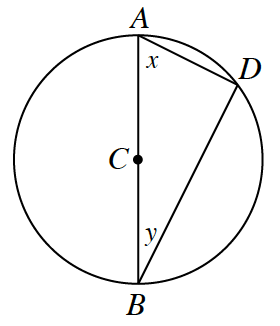### Home > CCG > Chapter 12 > Lesson 12.1.1 > Problem12-11

12-11.Use the diagram of $\odot C$ at right to answer the questions below.

1. If $m\angle x=28^\circ$, what is $m\overarc { A D }$?

$\angle D$ is a right angle. Why?

measure of arc $AD = 124^\circ$

2. If $AD = 5$ and $BD = 5\sqrt { 3 }$, what is the area of $\odot C$?

Use the Pythagorean Theorem to find the length of $AB$.
How can you use that length to find the area?

$25\pi \text{ un}^2$

3. If the radius of $\odot C$ is 8 and if $m \overarc { B D } = 100^\circ$, what is $BD$?

Draw radius $CD$. Use the fact that $\angle BCD=100^\circ$ and the Law of Sines to find $BD$.